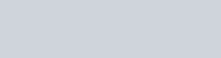A pole has to be erected at a point on the boundary of a circular park of diameter 13 meters in
Question:

A pole has to be erected at a point on the boundary of a circular park of diameter 13 meters in such a way that the difference of its distance from two diametrically opposite fixed gates A and B on the boundary is 7 meters. Is it the possible to do so? If yes, at what distances from the two gates should the pole be erected?

Solution:

Let $P$ be the required location on the boundary of a circular park such that its distance from gate $B$ is $=x$ metres that is $B P=x$ metres

Then, $A P=x+7$

In the right triangle ABP we have by using Pythagoras theorem

$A P^{2}+B P^{2}=A B^{2}$

$(x+7)^{2}+x^{2}=(13)^{2}$

$x^{2}+14 x+49+x^{2}=169$

$2 x^{2}+14 x+49-169=0$

$2 x^{2}+14 x-120=0$

$2\left(x^{2}+7 x-60\right)=0$

$x^{2}+7 x-60=0$

$x^{2}+12 x-5 x-60=0$

$x(x+12)-5(x+12)=0$

$(x+12)(x-5)=0$But, the side of right triangle can never be negative.

Therefore, $x=5$

Hence, $P$ is at a distance of 5 meters from the gate $B$.